## Communicating with Pocket Science Lab via USB and capturing and plotting sine waves

Design of PSLab combines the flexibility of Python programming language and the real-time measurement capability of micro-controllers.

PSLab, with its simple and open architecture allows users to use the tool for various measurements and to develop new experiments with simple functions written in python.

PSLab is interfaced and powered by USB port of the computer. For connecting external signals it has several input/output terminals as shown in the figure.#### Interfacing with the real world

Connecting to PSLab is as simple and straight forward as this…

```>>> from PSL import sciencelab
>>> I = sciencelab.connect()     #Returns None if device isn't found
# An example function that measures voltage present at the specified analog input
>>> print I.get_average_voltage('CH1')```

Various sensors can be connected to PSLab and data can be fetched with a simple python code as shown below…

```>>> from PSL.SENSORS import HMC5883L #A 3-axis magnetometer
>>> M = HMC5883L.connect()
>>> Gx,Gy,Gz = M.getRaw()
```

The module sciencelab.py contains all the functions required for communicating with PSLab hardware. It also contains some utility functions. The class ScienceLab() contains methods that can be used to interact with the PSLab. The connect() function returns an object of this class if PSLab hardware is detected.

The initialization process does the following

* connects to tty device

* loads calibration values.

```>>> from PSL import sciencelab
>>> I = sciencelab.connect()
>>> print I
<PSL.sciencelab.ScienceLab instance at 0x7fe9a7bf0e18>```

After initiating this class, its various function calls will allow access to all the features built into the device. Some examples showing the use of few function calls are given below…

#### Example 1: Capturing and plotting a sine wave

The function call used,

`capture1(self,ch,ns,tg,*args,**kwargs)`

Arguments

• ch  : Channel to select as input. [‘CH1′..’CH3′,’SEN’]
• ns  :  Number of samples to fetch. Maximum 10000
• tg   :  Time gap between samples in microseconds

Example Program

Connect WG1 to CH1 and run the following code.

```>>> from pylab import *
>>> from PSL import sciencelab
>>> I=sciencelab.connect()
>>> I.set_gain('CH1', 3) # set input CH1 to +/-4V range
>>> I.set_sine1(1000) # generate 1kHz sine wave on output W1
>>> x,y = I.capture1('CH1', 1000, 10) # digitize CH1 1000 times, with 10 usec interval
>>> plot(x,y)
>>> show()```

For running the script in IDE, one should define source code encoding, add this to the top of your script:

``# -*- coding: utf-8 -*-``

The output of the program is here…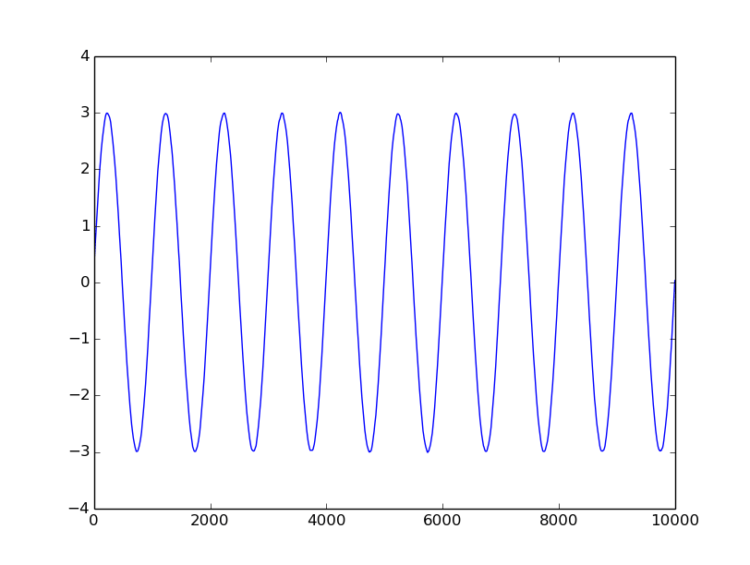#### Example 2 : Capturing two sine waves and plotting

The function call used,

`capture2(self,ns,tg,TraceOneRemap='CH1')`

Arguments

• ns :  Number of samples to fetch. Maximum 5000
• tg  :  Time gap between samples in microseconds
• TraceOneRemap :   Choose the analogue input for channel 1 (Like MIC OR SEN). It is connected to CH1 by default. Channel 2 always reads CH2.

Example Program

Connect WG1 to CH1, WG2 to CH2 and run the following code.

```# -*- coding: utf-8 -*-

from pylab import *
from PSL import sciencelab
I=sciencelab.connect()
I.set_gain('CH1', 2) # set input CH1 to +/-4V range
I.set_gain('CH2', 3) # set input CH2 to +/-4V range
I.set_sine1(1000) # generate 1kHz sine wave on output W1
I.set_sine2(1000) # generate 1kHz sine wave on output W2

x,y1,y2 = I.capture2(1600,1.75,'CH1')
plot(x,y1) #Plot of analog input CH1
plot(x,y2) #plot of analog input CH2
show()```

The output of the program is here…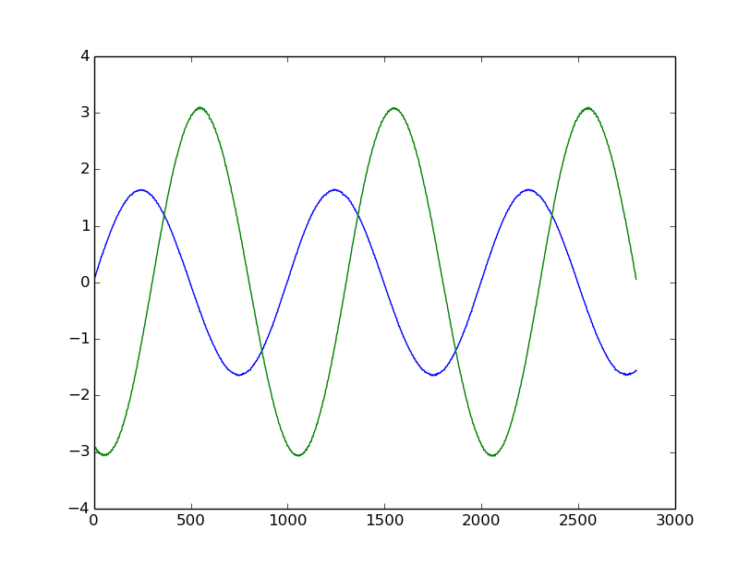#### Example 3 : Capturing four traces and plotting

The function call used,

`capture4(self,ns,tg,TraceOneRemap='CH1')`

Arguments

• ns:   Number of samples to fetch. Maximum 2500
• tg :   Time gap between samples in microseconds. Minimum 1.75uS
• TraceOneRemap :   Choose the analogue input for channel 1 (Like MIC OR SEN). It is connected to CH1 by default. Channel 2 always reads CH2.

Example Program

Connect WG1 to CH1, WG2 to CH2, SQR1 to CH3 and transducer mic to MIC (CH4) and run the following code.

```# -*- coding: utf-8 -*-

from pylab import *
from PSL import sciencelab
I=sciencelab.connect()
I.set_gain('CH1', 2) # set input CH1 to +/-4V range
I.set_gain('CH2', 3) # set input CH2 to +/-4V range
I.set_sine1(1000) # generate 1kHz sine wave on output W1
I.set_sine2(1000) # generate 1kHz sine wave on output W2
I.sqr1(2000,duty_cycle=50) # generate 1kHz square wave on output SQR1

x,y1,y2,y3,y4 = I.capture4(800,1.75)
plot(x,y1) #Plot of analog input CH1
plot(x,y2) #plot of analog input CH2
plot(x,y3) #plot of analog input CH3
plot(x,y4) #plot of analog input CH4 : MIC
show()```

The output of the program is here…##### Next To Do for GSoC-16

A detailed User manual and programmers manual with description of all function calls. ( Work in progress 🙂  )

## Features and Controls of Pocket Science Lab

Prerequisite reading:

PSLab is equipped with array of useful control and measurement tools. This tiny but powerful Pocket Science Lab enables you to perform various experiments and study a wide range of phenomena.

Some of the important applications of PSLab include a 4-channel oscilloscope, sine/triangle/square waveform generators, a frequency counter, a logic analyser and also several programmable current and voltage sources.

Add-on boards, both wired as well as wireless(NRF+MCU), enable measurement of physical parameters ranging from acceleration and angular velocity, to luminous intensity and Passive Infra-red. (Work under progress…)

As a reference for digital instruments a 12-MHz Crystal is chosen and a 3.3V voltage regulator is chosen for the analogue instruments. The device is then calibrated against professional instruments in order to squeeze out maximum performance.

Python based communication library and experiment specific PyQt4 based GUI’s make PSLab a must have tool for programmers, hobbyists, science and engineering teachers and also students.

PSLab is interfaced and powered by USB port of the computer. For connecting external signals it has several input/output terminals as shown in the figure.New panel design for PSLab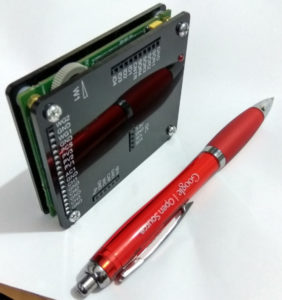#### Feature list for the acquisition and control :

• The most important feature of PSLab is a 4-channel oscilloscope which can monitor analog inputs at maximum of 2 million samples per second. Includes the usual controls such as triggering, and gain selection. Uses Python-Scipy for curve fitting.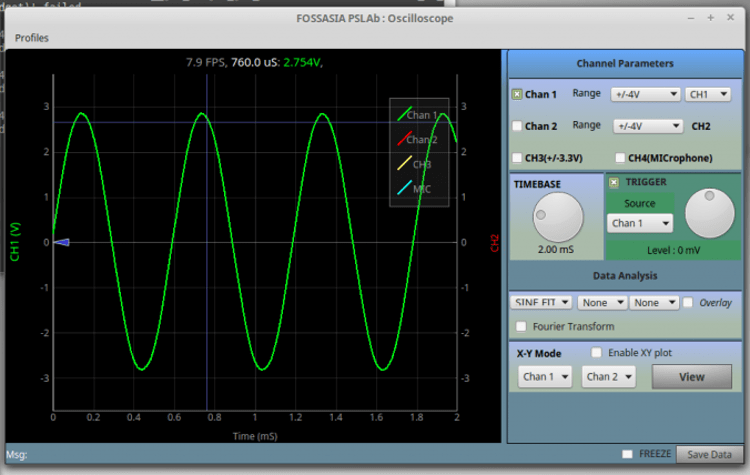PSLab Oscilloscope

Waveform Generators

• W1 : 5Hz – 5KHz arbitrary waveform generator. Manual amplitude control up to +/-3Volts
• W2 : 5Hz – 5KHz arbitrary waveform generator. Amplitude of +/-3Volts. Attenuable via software
• PWM : There are four phase correlated PWM outputs with maximum frequency 32MHz, 15nano second duty cycle, and phase difference control.

Measurement Functions

• Frequency counter tested up to 16 MHz.
• Capacitance Measurement. pF to uF range
• PSLab has several 12-bit Analog inputs (function as voltmeters) with programmable gains, and maximum ranges varying from +/-5mV to +/-16V.

Voltage and Current Sources

• 12-bit Constant Current source. Maximum current 3.3mA [subject to load resistance].
• PSLab has three 12-bit Programmable voltage sources/ +/-3.3V,+/-5V,0-3V . (PV1, PV2, PV3)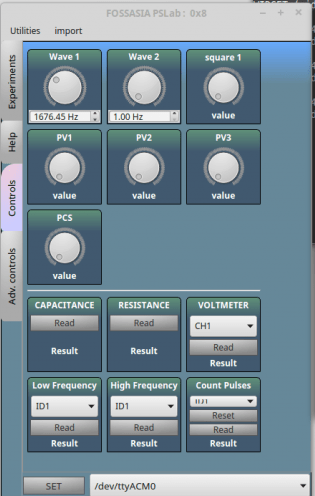Main Control Panel

Other useful tools

• 4MHz, 4-channel Logic analyzer with 15nS resolution.Voltage and Current Sources
• SPI,I2C,UART outputs that can be configured and controlled entirely through Python functions. (Work in progress…)
• On-board 2.4GHz transceiver for wireless data acquisition. (Work in progress..)
• Graphical Interfaces for Oscilloscope, Logic Analyser, streaming data, wireless acquisition, and several experiments developed that use a common framework which drastically reduces code required to incorporate control and plotting widgets.
• PSLab also has space for an ESP-12 module for WiFi access with access point / station mode.

Screen-shots of GUI apps.Advanced Controls with OscilloscopeWireless Sensors ( Work in progress…)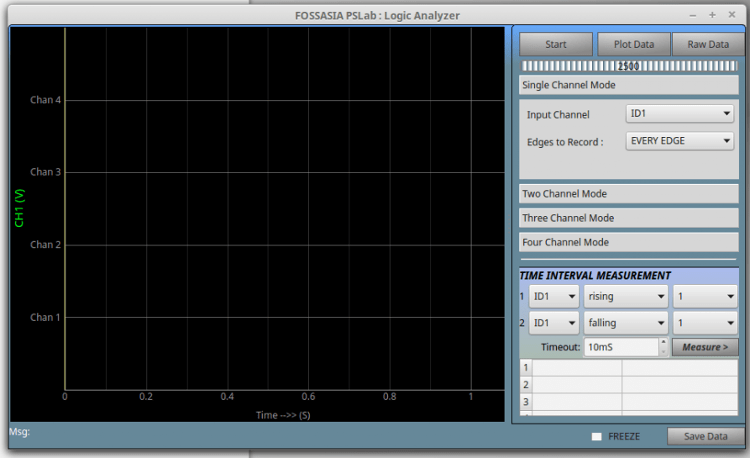Logic Analyzer

With all these features PSLab is taking a good shape and I see it as a potential tool that can change the way we teach and learn science. 🙂 🙂